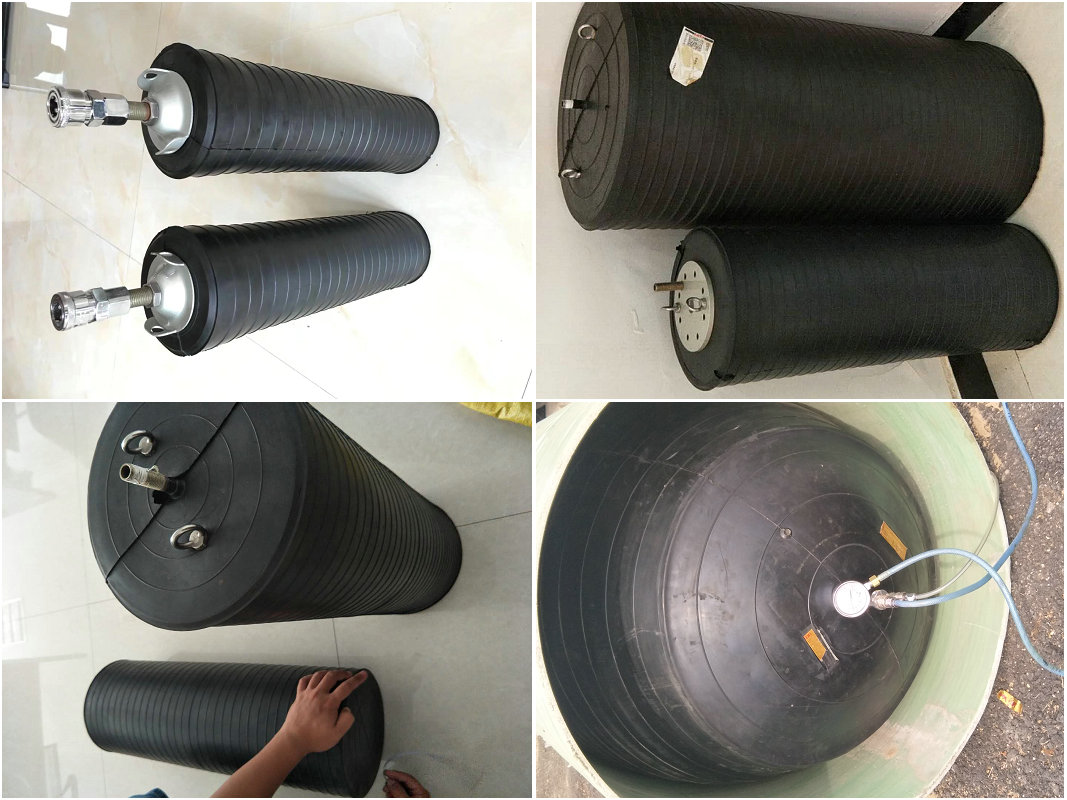+86-318-8538202

# FEET-OF-WATER-HEAD TO PSIG

HOW TO CALCULATE PIPELINE FORCES AND PRESSURES.

A. MEASURE THE INSIDE DIAMETER OF THE PIPE LINE IN INCHES.

B. DETERMINE THE MAXIMUM BACK PRESSURE.

Air pressure is measured in PSIG (Pounds Per Square Inch Gauge). Water pressure is calculated by determining the height of water column in feet above the center line of the pipe. Water height is multiplied by (0.43) to yield water pressure in PSIG. A FEET-OF-WATER-HEAD TO PSIG conversion table is also provided (see Table 1, next page).

C. CALCULATE THE PIPE AREA IN SQUARE INCHES. Area of a circle (square inches) = [Diameter (in inches) x Diameter (in inches) x 3.1416 (pi)] / 4.

D. CALCULATE THE FORCE THE PLUG MUST WITHSTAND. FORCE = PIPE AREA (in square inches) x PRESSURE (PSIG).

EXAMPLE: A plug must hold a (10) foot column of water above the center line of a 60” diameter pipe.

PIPE AREA = [60” (dia) x 60” (dia) x 3.1416 (pi)]/ 4 = 2,827 square inches.

PRESSURE = 10 Feet of Water x 0.43 PSIG/Foot = 4.3 PSIG

PIPE AREA (2,827 square inches) x PRESSURE (4.3 PSIG) = FORCE = 12,157 pounds.

Following is the force the plug must withstand

 WATER HEIGHT Back Pressure 3m 0.3bar 6m 0.6bar 9m 0.9bar 10m 1bar 12m 1.2bar 15m 1.5bar 20m 2bar

CONVERSION TABLE

FEET-OF-WATER-HEAD TO PSIG

(Pounds Per Square Inch Gauge)Inquiry for Our Product

Zaoqiang Dacheng Rubber Co., Ltd.
E-mail: info@marineairbags.com

When you contact us, please provide your detail requirements.
That will help us give you a valid quotation.

1.Products List
Rubber Pipe Plugs Inflatable Rubber Mandrel Marine Airbag